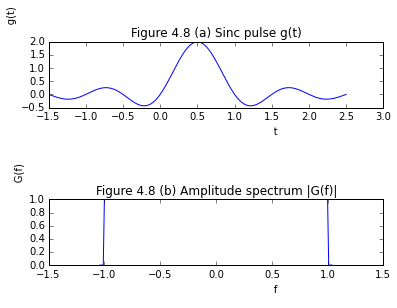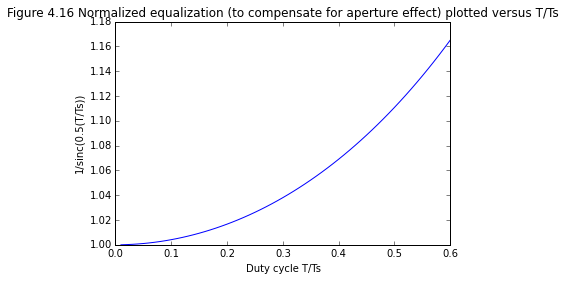# Chapter 4 Sampling Process¶

## Example4.1 page 164¶

In :
from __future__ import division
from numpy import arange,ones,sinc
%matplotlib inline
from matplotlib.pyplot import plot,xlabel,ylabel,show,title,subplot

t = arange(-1.5,0.01+2.5,0.01)
g = [2*sinc(2*tt-1) for tt in t]
print 'Aliasing error cannot exceed max|g(t)| = ',max(g)
f = arange(-1,0.01+1,0.01)
G = [0,0,0,0]+[xx for xx in ones(len(f))]+[0,0,0,0]
f1 = arange(-1.04,0.01+1.04,0.01)
subplot(3,1,1)
plot(t,g)
xlabel('                                      t')
ylabel('                                      g(t)')
title('Figure 4.8 (a) Sinc pulse g(t)')
subplot(3,1,3)
plot(f1,G)
xlabel('                                      f')
ylabel('                                      G(f)')
title('Figure 4.8 (b) Amplitude spectrum |G(f)|')
show()

Aliasing error cannot exceed max|g(t)| =  2.0## Example4.3 page 165¶

In :
from __future__ import division
from numpy import arange,ones,sinc,pi,sin
%matplotlib inline
from matplotlib.pyplot import plot,xlabel,ylabel,show,title,subplot

T_Ts = arange(0.01,0.01+0.6,0.01)
#E = 1/(sinc_new(0.5*T_Ts))#
E=
for i in range(1,len(T_Ts)):
E.append(((pi/2)*T_Ts[i])/(sin((pi/2)*T_Ts[i])))

plot(T_Ts,E)
xlabel('Duty cycle T/Ts')
ylabel('1/sinc(0.5(T/Ts))')
title('Figure 4.16 Normalized equalization (to compensate for aperture effect) plotted versus T/Ts')
show()